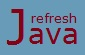# Java Nested Loops

Java programming allows programmers to place one loop statement inside body of another loop statement. For example, a `for` loop can be placed inside other `for` loop, a `while` loop can be placed inside other `while` loop, a `while` loop can be place inside other `for` loop etc. The process of placing one loop inside other loop is called nesting, and such loops are called as nested loops. The syntax of nested loops are:

``` `for`(init; condition; inc/dec)                  `while`(condition1)
{                                               {
`// outer loop code`                                 `// outer loop code`
`for`(init; condition; inc/dec)                   `while`(condition2)
{                                               {
`// inner loop code`                                  `// inner loop code`
. . . . . .                                     . . . . . .
. . . . . .                                     . . . . . .
}                                               }
`// outer loop code`                                  `// outer loop code`
}                                               }```

• For every iteration of outer loop, inner loops completes all it's iteration.
• A single loop can contain multiple loops at same level inside it.
• It's perfectly legal to place, `for` inside `while`, `while` inside `for`, `for` and `while` in the same level etc.

### How many times I can do nesting of loops ?

The process of repeating the nesting can be done any number of times.

### Nested for loop program in Java

```` class` NestedForLoop
{
`public static void` main(String [] args)
{
`for`(`int` i=1;i<=3;i++)
{
System.out.println(`"Outer loop iteration i =  "`+i);
`for`(`int` j=1;j<=3;j++)
{
System.out.println(`"j =  "`+j);
}
}
}
}```

Output:

Outer loop iteration i = 1
j = 1
j = 2
j = 3
Outer loop iteration i = 2
j = 1
j = 2
j = 3
Outer loop iteration i = 3
j = 1
j = 2
j = 3

### Nested while loop program in Java

```` class` NestedWhileLoop
{
`public static void` main(String [] args)
{
`int` i=0,j=0;
`while`(i<3)
{
j=0;
`while`(j<3)
{
System.out.print(i+`""`+j+ `"  "`);
j++;
}
System.out.println(`""`);
i++;
}
}
}```

Output:

``` 00  01  02
10  11  12
20  21  22  ```
★★★
• Use nested loops whenever actually needed, as nesting increases the complexity.
• Apply the conditions in nested loops properly, sometime incorrect conditions results as infinite loops.
• Nested loops are helpful when printing some pattern in multi-dimension.